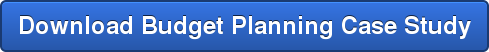Contact us - 262-780-0962

### Subscribe to Email Updates

INTRODUCTION:

In the previous post, we described the first couple of steps required for setting up multiple linear regression models for prediction. These steps focused mainly on exploring the predictors or variables in the data set that would influence the outcome. It was also mentioned that wrapper type feature selection methods such as forward selection or backward elimination are usually used to select the variables which will go into the model. In this article, we will look at how one of these methods can be employed to build a model and once the model is built how to quantify the model performance. In particular, we will explain the differences between using the adjusted R2 and standard error of the regression estimate to evaluate modelperformance.

### Step 3: Building the regression model

This is perhaps the easiest of the steps given the fact that so many powerful tools exist to simplify the job of selecting the right attributes or variables that go into the model. For example, to use backward elimination using R, we first build the full model using `glm()` and make the following call to the `step()` function to obtain the final model.

`finalModel <- step(fullModel, direction="backward", trace=FALSE)`

In RapidMiner, we would use the `Backward Elimination` operator and nest the `Linear Regression` operator inside it (see these articles). These feature selection steps utilize the Akaike Information Criterion (AIC) to determine when to stop adding or removing attribues.

For smaller dimensions (fewer predictors), it may be better to do a manual "feature selection" and using ANOVA. We first build a model with one variable (possibly the one with the highest correlation coefficient to the outcome) and then add the next strongly correlated variable. We can then run an ANOVA to determine if the null hypothesis (which states "adding the second variable is not significant") can be rejected at a particular confidence level (usually 0.005).

`#ANOVA for Model 1 and Model 2 - Significance of adding a second variable.`
`x <-anova(model1, model2)`
`x\$Pr`

If the output of the second statement is below the cutoff confidence level, then we continue to add the next variables. If not we can skip adding the second variable and so on.

The main job of the analyst is in interpreting the results of the model fit and making a decision on whether the model is good enough or needs additional iterations and then potentially explain to non-technical users his/her choice. For an analyst who is trying to explain to business users the reason why the model was chosen, AIC or ANOVA may be hard to explain. A better option for this is to use simple tools such as adjusted R2 and standard error (SE) of the estimate.

### Step 4: Model Performance: R2 and Standard Error (SE) of the estimate

Simply stated, R2 compares the predicted Y's to the actual Y's. But before taking this ratio, it subtracts the actual Y-average from each term, and does the summation. R2 is essentially the square of the correlation between x and y, for a simple one variable-one outcome problem.

But there are 3 problems with using only R2 as an indicator of model fit:

1. Reducing the number of data points tends to inflate R2
2. Increasing the number of variables tends to inflate R2. This is addressed by using what is called the Adjusted R2.
3. Finally, using a no-intercept model also inflates R2. (see this StackExchange discussion for more details on why).

For these reasons, it may sometimes be better to use the SE of the estimate. SE is essentially the standard deviation of the error (residuals) of the fitted model. Recall that the residual is simply given by (Actual value of outcome – Fitted value of outcome). SE may be a better indicator of model fit and performance because it is measured in the same units as the predicted variable (for example, in \$ or number of units etc). Furthermore, we can (under conditions of normal distribution), estimate confidence intervals for the predicted/fitted outcome values. For example, 68% of all fitted (predicted) values < 1*SE and 95% of all fitted (predicted) values < 2*SE. It is far more intuitive to explain to a business user that "on a predicted price of \$35, we can state with 95% confidence that the actual price will be within +/- \$3 of the predicted price if we use model A" and so on.

Now that we have built several models and selected the best performing one (along with giving business reasons why), we can get into a little more detail on the statistical understanding of the regression model itself, including p-values, residual analysis, inference tests and finally interpreting the coefficients. This will be described in the next article. In the meantime download a case study to learn more about business use cases.Topics: multiple linear regression

### Most Popular